Question
only need questions B,C, D, and E done.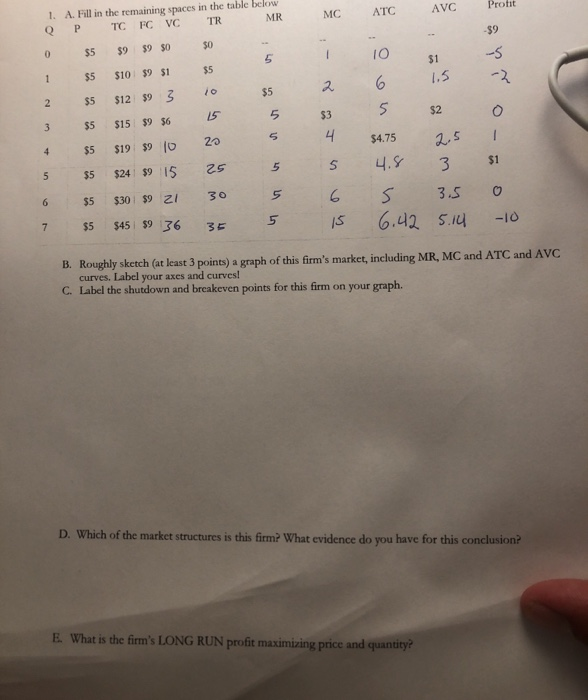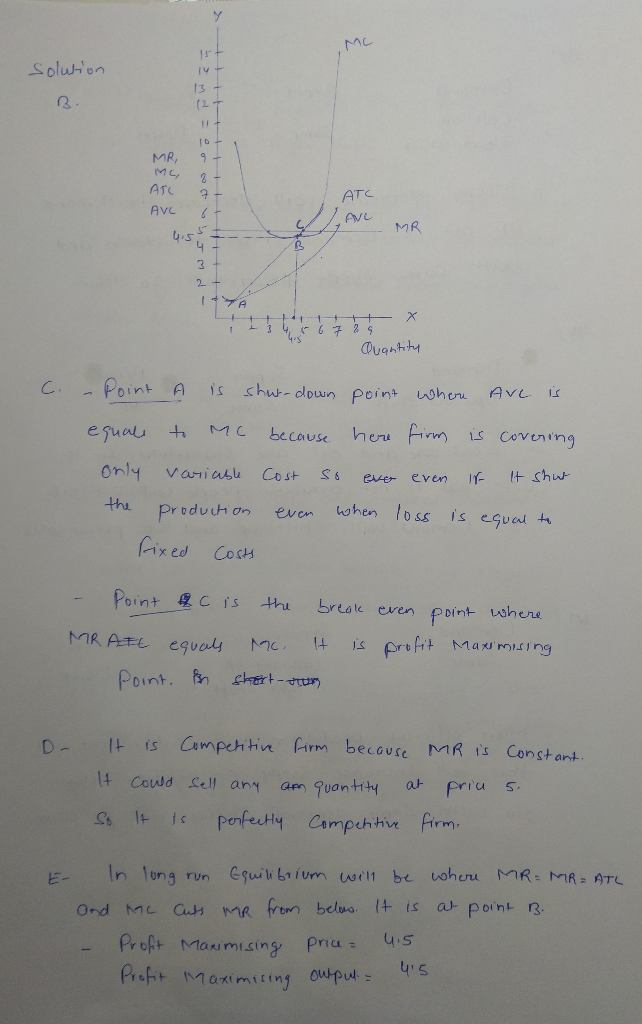#### Earn Coins

Coins can be redeemed for fabulous gifts.

Similar Homework Help Questions
• ### MR MC Profit ATC 1. A. Fill in the remaining spaces in the table below QP...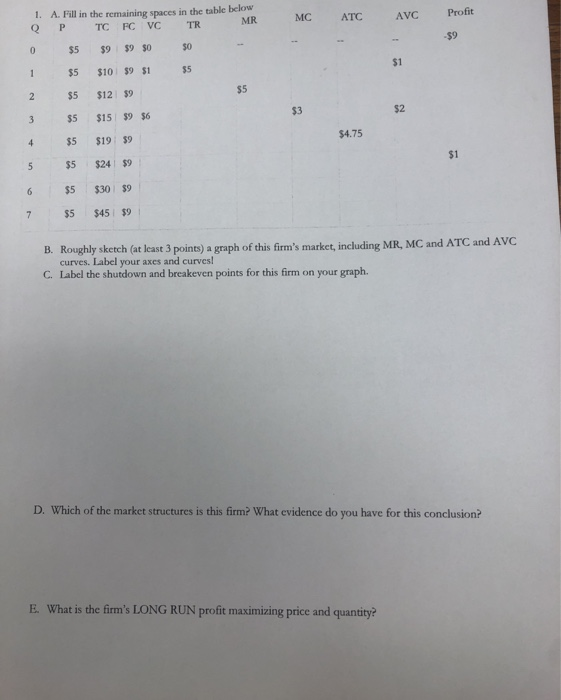MR MC Profit ATC 1. A. Fill in the remaining spaces in the table below QP TCFCVC TR 0 \$5 \$9 \$9 \$0 \$0 1 \$5 \$10 \$9 \$1 \$5 \$5 \$12 \$5 \$15 \$9 \$6 4 \$5 \$1959 \$5 \$24 \$9 \$5 \$30 59 \$5 \$45 \$9 \$4.75 B. Roughly sketch (at least 3 points) a graph of this firm's market, including MR, MC and ATC and AVC curves. Label your axes and curves! C. Label the shutdown and...

• ### Use Figure 1 to answer questions 1-2. Figure 1 Dollars ATC Output 100 150 180 210...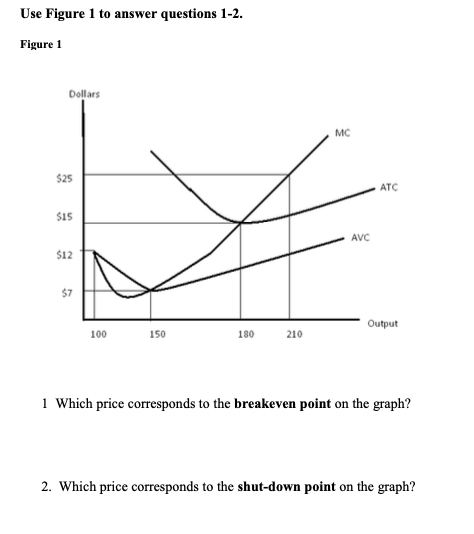Use Figure 1 to answer questions 1-2. Figure 1 Dollars ATC Output 100 150 180 210 1 Which price corresponds to the breakeven point on the graph? 2. Which price corresponds to the shut-down point on the graph? Use Figure 2 to answer questions 3-5. Figure 2 Dollars MC ATC AVC Output 100 150 180 210 Assume that the market price is \$25. 3. Should the firm produce or shutdown? Why? 4. What is the firm's profit maximizing output level?...

• ### 2. A. Fill in the table below. AC Quantity Total Cost Total Profit PPU TR \$20 B. If this industry only has one firm...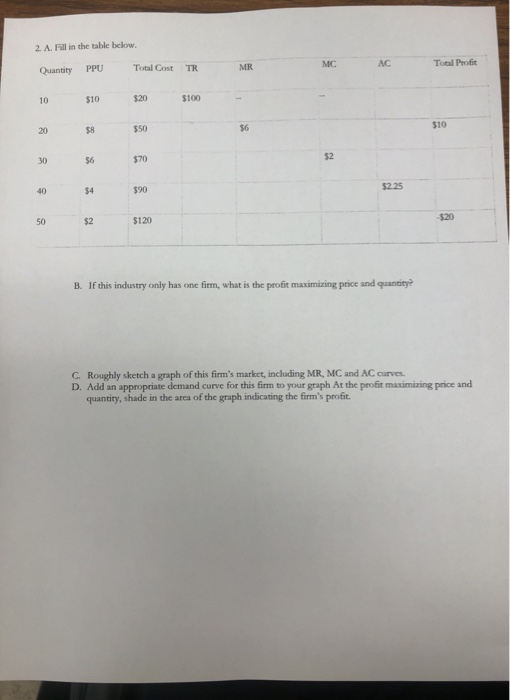2. A. Fill in the table below. AC Quantity Total Cost Total Profit PPU TR \$20 B. If this industry only has one firm, what is the profit maximizing price and quantity? C. Roughly sketch a graph of this firm's market, including MR, MC and AC curves. D. Add an appropriate demand curve for this firm to your graph At the profit maximizing price and quantity, shade in the area of the graph indicating the firm's profit.

• ### Consider a competitive firm with total costs given by TC(q) = 100 + 10q + q...

Consider a competitive firm with total costs given by TC(q) = 100 + 10q + q 2 The firm faces a market price p = 50. (d) Find the profit-maximizing level of output q^*. At this level of output, what are TR, TC, ATC, and π? (e) Graph the ATC, AVC, MC, and MR curves in a single graph, and indicate the profit-maximizing level of output. If there are profits, shade the region corresponding to profit and label it.

• ### Consider a competitive rm with total costs given by TC(q) = 100 + 10q + q^2,...

Consider a competitive rm with total costs given by TC(q) = 100 + 10q + q^2, The firm faces a market price p = 50. (a) Write expressions for total revenue TR and marginal revenue MR as functions of output q. (b) Write expressions for average total cost ATC, average variable cost AVC, and marginal cost MC as functions of output q. (c) For what value of output is ATC minimized? (d) Find the profit maximizing level of output q...

• ### 3. Given the data below, compute TR, TC, TFC, TVC, ATC, AVC, AFC, MR, MC, AR,...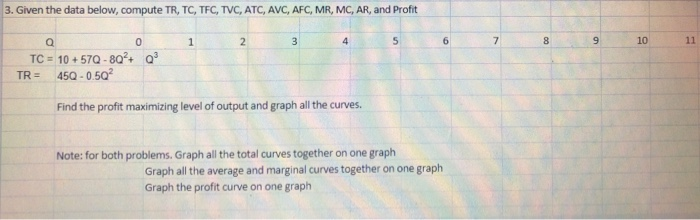3. Given the data below, compute TR, TC, TFC, TVC, ATC, AVC, AFC, MR, MC, AR, and Profit 1 2 3 6 7 8 9 10 11 0 TC = 10 +570 - 80+ TR = 452 - 0 50. Find the profit maximizing level of output and graph all the curves. Note: for both problems. Graph all the total curves together on one graph Graph all the average and marginal curves together on one graph Graph the profit curve...

• ### 1) A perfectly competitive firm faces the following Total revenue, Total cost and Marginal cost functions:...

1) A perfectly competitive firm faces the following Total revenue, Total cost and Marginal cost functions: TR = 10Q TC = 2 + 2Q + Q2 MC = 2 + 2Q At the level of output maximizing profit , the above firm's level of economic profit is                                                                                                           A) \$0 B) \$4 C) \$6 D) \$8 *Additional information after I did the math: The price this firm charges for its product is \$10, the level of output maximizing profit is 4...

• ### show all steps and formulas VC A product in a perfectly competitive market is \$6. Assume...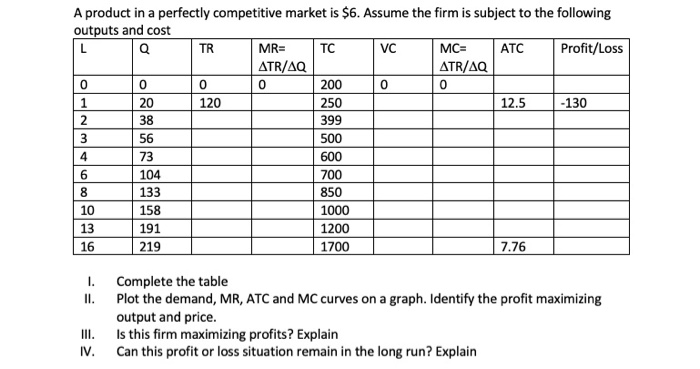show all steps and formulas VC A product in a perfectly competitive market is \$6. Assume the firm is subject to the following outputs and cost MR= TC MC= ATC Profit/Loss ATR/AQ ATR/AQ 200 250 12.5 -130 399 500 600 700 850 158 1000 191 1200 16 219 1700 7.76 II. Complete the table Plot the demand, MR, ATC and MC curves on a graph. Identify the profit maximizing output and price. Is this firm maximizing profits? Explain Can this...

• ### The market price is p=50 3. Consider a competitive firm with total costs given by TC(q)...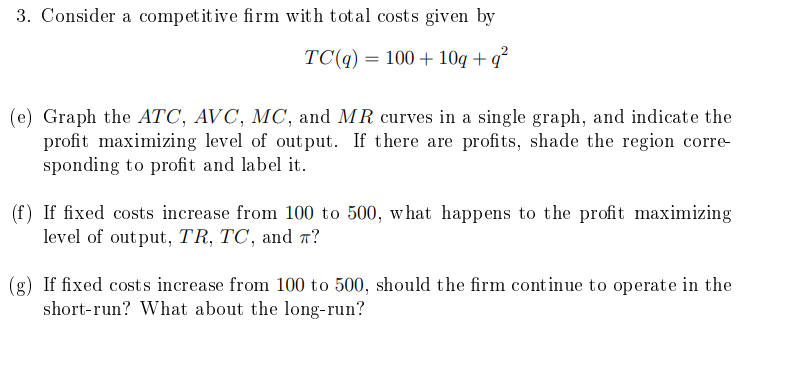The market price is p=50 3. Consider a competitive firm with total costs given by TC(q) = 100 + 10q+q? (e) Graph the ATC, AVC, MC, and MR curves in a single graph, and indicate the profit maximizing level of output. If there are profits, shade the region corre- sponding to profit and label it. (f) If fixed costs increase from 100 to 500, what happens to the profit maximizing level of output, TR, TC, and a? (g) If fixed...

• ### The graph to the right depicts the average cost curves and the marginal cost curve for...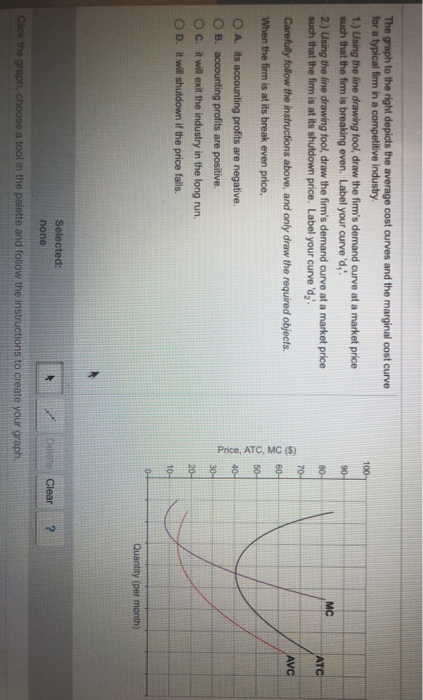The graph to the right depicts the average cost curves and the marginal cost curve for a typical firm in a competitive industry. 1.) Using the line drawing fool, draw the firm's demand curve at a market price such that the firm is breaking even. Label your curved, 2.) Using the line drawing tool, draw the firm's demand curve at a market price such that the firm is at its shutdown price. Label your curved, Carefully follow the instructions above,...